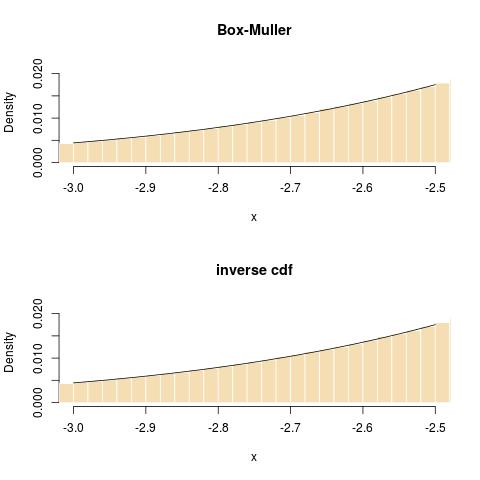Want to share your content on R-bloggers? click here if you have a blog, or here if you don't.

Another Cross Validated forum question that led me to an interesting (?) reconsideration of certitudes! When simulating from a normal distribution, is Box-Muller algorithm better or worse than using the inverse cdf transform? My first reaction was to state that Box-Muller was exact while the inverse cdf relied on the coding of the inverse cdf, like qnorm() in R. Upon reflection and commenting by other members of the forum, like William Huber, I came to moderate this perspective since Box-Muller also relies on transcendental functions like sin and log, hence writing$X=\sqrt{-2\log(U_1)}\,\sin(2\pi U_2)$

also involves approximating in the coding of those functions. While it is feasible to avoid the call to trigonometric functions (see, e.g., Algorithm A.8 in our book), the call to the logarithm seems inescapable. So it ends up with the issue of which of the two functions is better coded, both in terms of speed and precision. Surprisingly, when coding in R, the inverse cdf may be the winner: here is the comparison I ran at the time I wrote my comments

> system.time(qnorm(runif(10^8)))
sutilisateur     système      écoulé
10.137           0.120      10.251
> system.time(rnorm(10^8))
utilisateur     système      écoulé
13.417           0.060      13.472


However re-rerunning it today, I get opposite results (pardon my French, I failed to turn the messages to English):

> system.time(qnorm(runif(10^8)))
utilisateur     système      écoulé
10.137       0.144      10.274
> system.time(rnorm(10^8))
utilisateur     système      écoulé
7.894       0.060       7.948
`

(There is coherence in the system time, which shows rnorm as twice as fast as the call to qnorm.) In terms, of precision, I could not spot a divergence from normality, either through a ks.test over 10⁸ simulations or in checking the tails:Filed under: Books, Kids, R, Statistics, University life Tagged: Box-Muller algorithm, cross validated, inverse cdf, logarithm, normal distribution, qnorm()